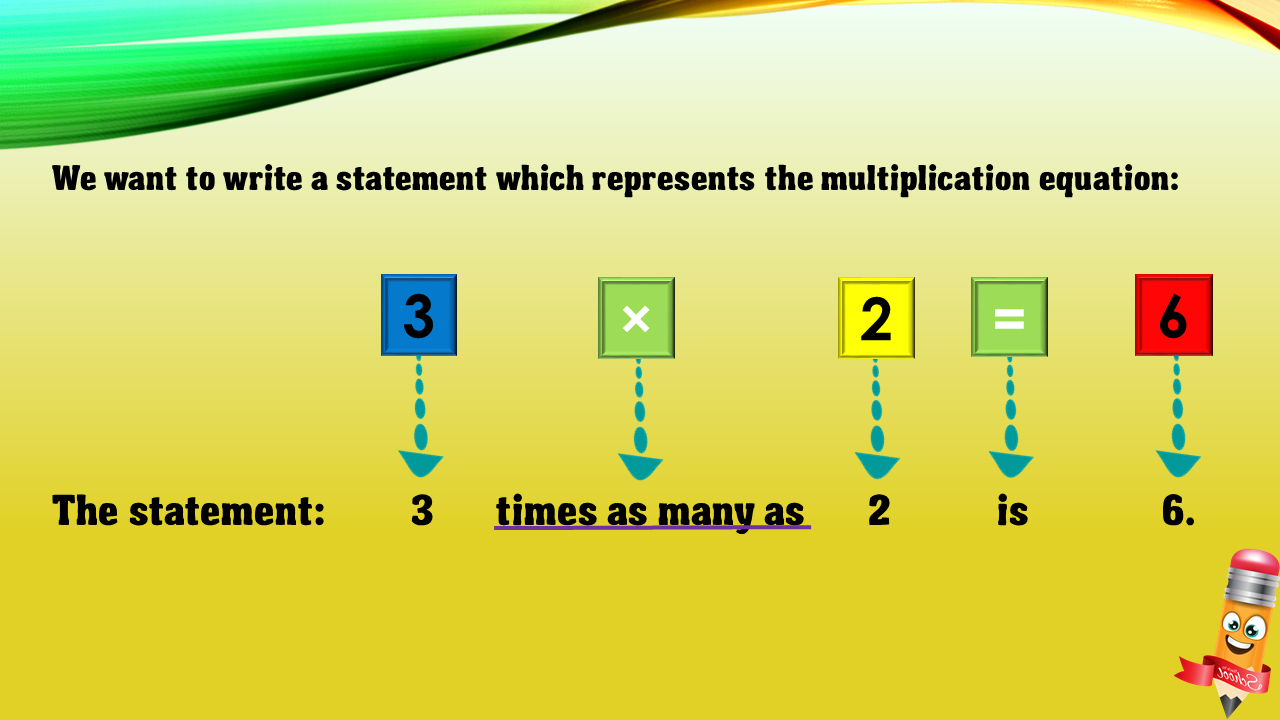1
visibility

Choose one statement which represents the equation:
3 × 2 = 6 ?

• A

3 more than 2 is 6.

• B

2 less than 6 is 3.

• C

2 times as many as 6 is 3.

• D

3 times as many as 2 is 6.